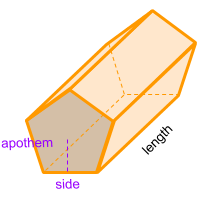Home » Polyhedra » Prisms » Pentagonal Prism

# Volume of a Pentagonal PrismVolume =
5 × Sidep × Apothemp/2
× Length

## Volume calculator for a pentagonal prism

Enter a Side and the Apothem of the pentagon that forms one of the bases of the pentagonal prism along the length of the prism to get the volume automatically.

If you don't know the value of the apothem you can enter a side of the pentagon that forms one of the bases of the pentagonal prism along the length of the prism to get the value automatically.

## Description, how many faces, edges and vertices are there in a pentagonal prism

The pentagonal prism, has 7 faces, 2 of these faces are equal and parallel pentagons and form the bases at the ends of the shape. Another 5 faces are parallelograms. It has 15 edges and 10 vertices. If a cross section is made in any part of its length, it always maintains the shape of a pentagon.

## Formula for the volume of a pentagonal prism

To calculate the volume of a pentagonal prism, the formula for all prism can be used, where the area of ​​the base is multiplied by the length of the prism. In this case, the base of the pentagonal prism is a pentagon, therefore, the area of ​​the pentagon needs to be a known value.

To calculate the area of ​​the pentagon (base area), multiply the perimeter of the pentagon by its apothem and divide in two. Finally to get the volume of the prism, multiply the result by the length.

Remember that the apothem is the distance from the center of the polygon to the middle of one of its sides. And also remember that the perimeter of a pentagon is the sum of all its sides or the side multiplied by 5. You can also use the online calculator to calculate the volume of the pentagonal prism automatically.

Volume =
5 × Sidep × Apothemp/2
× Length

Formula explanation and alternative formula:

The formula to calculate the volume of prism is always the same:

Volume prism = Area base × Length

In this case, the area of the base of the pentagonal prism is a pentagon:

Area base = Area pentagon =
Perimeter × Apothem/2

To calculate the perimeter:

Perimeter = 5 × Side

Replacing the perimeter formula in the formula shown above, then you get the main formula.

To get the volume of a pentagonal prism without knowing the apothem:

Apothem =
side/2•tan(α÷2)
, with
α =
360°/5

Therefor, another way to get the volume of a pentagonal prism without knowing the apothem is:

Volume =
5 × side ² / 4 × Tan(36°)
× length

Share